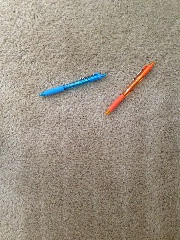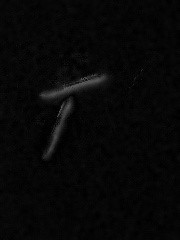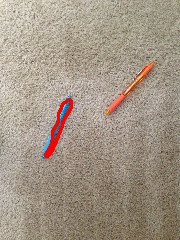Search

# How to track objects with stationary background?

Like I had promised in my previous blog, detecting circular objects, I am going to talk about one of the methods of tracking objects using computer vision. When I was in my undergrad school, I read a few research papers on Object Tracking and applied some really cool techniques to track ants. The research particularly was focused towards behavior analysis. It was fun and pretty challenging. The challenge mainly came from the fact that we couldn't predict the path an ant would traverse. Yup, that is very difficult. The speed at which it travels makes it even more difficult. A fun fact: I recently read that an ant can travel the circumference of earth in its lifetime.

Let's come back to tracking. So, today we will talk about two new functions in OpenCV and how we can use them in determining object movement and hence, tracking. For this tutorial we will be considering a scene with a stationary background. The images are going to be sequential, in time series. We will track the position of the object in the sequential frames.

I have selected these images for the tracking and analysis:To start with, let us consider that we do not have knowledge about which object is going to be moving in this image. It is pretty easy when no objects are in the frame and one appears from nowhere. But here, let us deal with a situation where one of these objects are going to be moving but we don't know which.

Since the background is stationary we will be using background subtraction to subtract the unnecessary, here stationary, information from two sequential images. The function that OpenCV provides for this purpose is absdiff.

`destination_image = cv2.absdiff(image1, image2)`

cv2.absdiff is a function which helps in finding the absolute difference between the pixels of the two image arrays. By using this we will be able to extract just the pixels of the objects that are moving. To use cv2.absdiff we will need to convert our images to grayscale(grayscale is a range of shades of gray ranging from black to white).

In the process of converting the images from color to grayscale, let's do some preprocessing to reduce noise. We'll perform preprocessing by applying a bilateral filter. It can be done with cv2.bilateralFilter:

```def preprocess_image(image):
bilateral_filtered_image = cv2.bilateralFilter(image, 7, 150, 150)
gray_image = cv2.cvtColor(bilateral_filtered_image, cv2.COLOR_BGR2GRAY)
return gray_image```Once we preprocess both the images, we can subtract the second sequential image from the first using cv2.absdiff

`image_sub = cv2.absdiff(preprocessed_image1, preprocessed_image2)`Now we're left with the two positions that the blue pen had over time. This is good, but it is not enough to give us a proper outline of an object. A little post processing of the image can help us in determining the outline of an object.

Before we do that, let's first apply a morphological operation. Here we will first dilate and then erode the image which is called a “close” operation. We shall then threshold the image to make the object more prominent. To remove the small objects in the image we'll proceed with a median filter which helps to remove 'salt and pepper' noise. Combining those and turning it in to code we get:

```kernel = numpy.ones((5,5),numpy.uint8)
close_operated_image = cv2.morphologyEx(image, cv2.MORPH_CLOSE, kernel)
_, thresholded = cv2.threshold(close_operated_image, 0, 255, cv2.THRESH_BINARY + cv2.THRESH_OTSU)

median = cv2.medianBlur(thresholded, 5)```Next we'll find the contours of the objects in the image. This technique gives us just the object which has moved.

```_, contours, _ = cv2.findContours(median, cv2.RETR_TREE, cv2.CHAIN_APPROX_SIMPLE)
cv2.drawContours(image, contours, -1, (100, 0, 255),2)```At this point we have an object which has moved. We've got a good outline of the object. We do not know which contour corresponds to which frame. We can match up the captured motion with our time sequence frame-by-frame. We find all the contours in the first frame and match the contour from this subtracted image to find the position of the object in the first frame. Once that is known, we can say that the second contour in the subtracted image is that of the object’s new position in the current frame.

Let's find the contours of the objects in the first frame. I'll use a new concept called 'orientation of the contours', in order to match the contours from first frame and current frame.

The function in OpenCV which helps find the orientation of a contour is cv2.fitEllipse:

`_, _, angle = cv2.fitEllipse(contour)`

cv2.fitEllipse gives the rotated rectangle or ellipse in which the contour is inscribed. The output of the function gives the angle at which the ellipse is with respect to the x-axis. We use this angle to match the contours of the first frame to the current frame. Any contours with angles that are close in value - typically difference less than 1 degree - are the same objects.

So we get the position of the object in frame 1:We therefore say that the other contour in the subtracted image is the position of the object in the current frame. We can use this position information to further do frame subtractions of the resulting images and track the objects.## Where do I use this?

If you are interested in tracking objects realtime with stationary background, this is the simplest method with tracking real-time. Object tracking is very famous in applications that involve detecting the speed of an object, especially of a vehicle. In the 3D printing industry one of the cool areas I know where object tracking is used is for capturing 3D models and then creating them virtually. To start with, you can integrate a camera to capture sequential frames and apply this algorithm to track the path of an insect, or a toy. Its fun. That's how I started learning to implement object tracking.

I hope you found this useful. Please share any tips to make this better.

4,883 views1 comment

## Recent Posts

See All

At Authentise we use databases quite a bit. That's not really all that odd, most web technologies need some way of persisting state long term and a database does just that. We like PostgresQL. There's

Arrows => Arrows are a shorthand for function. Old way: users.forEach(function(user) { // do something with the user }); New way: users.forEach(user => { // do something with the user }) Impor